Next: McCullough's Formula Up: Gravitational Potential Theory Previous: Potential Outside a Uniform

# Rotational Flattening

Let us consider the equilibrium configuration of a self-gravitating spheroid, composed of uniform density incompressible fluid, which is rotating steadily about some fixed axis. Let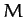be the total mass,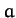the mean radius,the ellipticity, andthe angular velocity of rotation. Furthermore, let the axis of rotation coincide with the axis of symmetry, which is assumed to run along the-axis.

Let us transform to a non-inertial frame of reference which co-rotates with the spheroid about the-axis, and in which the spheroid consequently appears to be stationary. From Chapter 7, the problem is now analogous to that of a non-rotating spheroid, except that the surface acceleration is written, where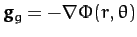is the gravitational acceleration, andthe centrifugal acceleration. The latter acceleration is of magnitude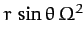, and is everywhere directed away from the axis of rotation (see Figure 40 and Chapter 7). The acceleration thus has components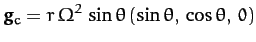(912)

in spherical polar coordinates. It follows that, where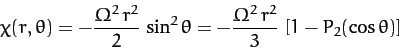(913)

can be thought of as a sort of centrifugal potential. Hence, the total surface acceleration is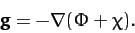(914)

As before, the criterion for an equilibrium state is that the surface lie at a constant total potential, so as to eliminate tangential surface forces which cannot be balanced by internal pressure. Hence, assuming that the surface satisfies Equation (901), the equilibrium configuration is specified by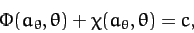(915)

where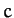is a constant. It follows from Equations (911) and (913) that, to first-order inand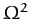,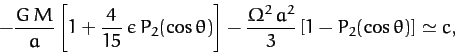(916)

which yields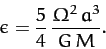(917)

We conclude, from the above expression, that the equilibrium configuration of a (relatively slowly) rotating self-gravitating mass distribution is an oblate spheroid: i.e., a sphere which is slightly flattened along its axis of rotation. The degree of flattening is proportional to the square of the rotation rate. Now, from (901), the mean radius of the spheroid is, the radius at the poles (i.e., along the axis of rotation) is, and the radius at the equator (i.e., perpendicular to the axis of rotation) is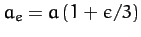--see Figure 40. Hence, the degree of rotational flattening can be written(918)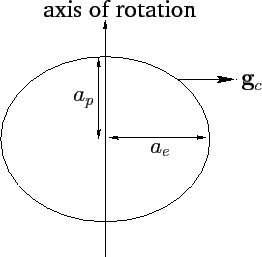Now, for the Earth,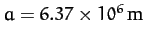,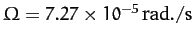, and. Thus, we predict that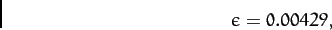(919)

corresponding to a difference between equatorial and polar radii of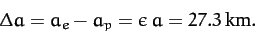(920)

In fact, the observed degree of flattening of the Earth is, corresponding to a difference between equatorial and polar radii of. The main reason that our analysis has overestimated the degree of rotational flattening of the Earth is that it models the terrestrial interior as a uniform density incompressible fluid. In reality, the Earth's core is much denser than its crust (see Exercise 12.1).

For Jupiter,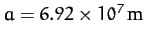,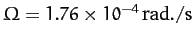, and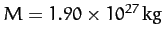. Hence, we predict that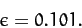(921)

Note that this degree of flattening is much larger than that of the Earth, due to Jupiter's relatively large radius (about 10 times that of Earth), combined with its relatively short rotation period (about 0.4 days). In fact, the polar flattening of Jupiter is clearly apparent from images of this planet. The observed degree of polar flattening of Jupiter is actually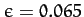. Our estimate ofis probably slightly too large because Jupiter, which is mostly gaseous, has a mass distribution which is strongly concentrated at its core (see Exercise 12.1).Next: McCullough's Formula Up: Gravitational Potential Theory Previous: Potential Outside a Uniform
Richard Fitzpatrick 2011-03-31